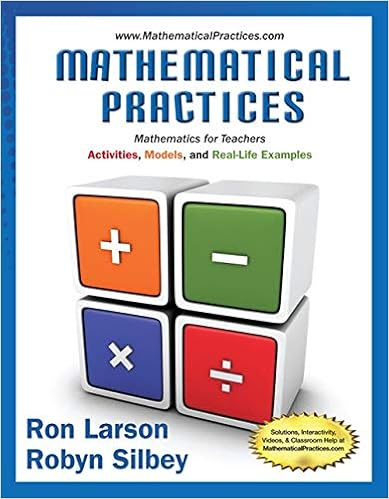# Week 7 Feedback Practice Q5 Problems ANS 2018.pdf - UMUC...

• Notes
• rhernandez77
• 7

This preview shows page 1 - 3 out of 7 pages.

##### We have textbook solutions for you!
The document you are viewing contains questions related to this textbook.The document you are viewing contains questions related to this textbook.
Chapter 10 / Exercise 20
Mathematical Practices, Mathematics for Teachers: Activities, Models, and Real-Life Examples
LarsonExpert Verified
UMUC Week 7 Math 009 Quiz 5 Feedback Practice ANS Winter 2018 Professor: Dr. Ty Allen Name________________________________ Instructions: The quiz is worth 50 points. There are 10 problems, each worth 5 points. Your score on the quiz will be converted to a percentage and posted in your assignment folder with comments. This quiz allows open bookand open notes, and you may take as long as you like on it provided that you submit the quiz no later than the due date posted in our course schedule of the syllabus. You may refer to your textbook, notes, and online classroom materials, Please remember to show ALL of your work on every problem. Read the basic rules for showing work posted in our LEO classroom BEFORE you start working on the quiz. 1) Graph the linear equation by finding and plotting the intercepts. Show all work and write each intercept as an ordered pair. You may draw your graph on the grid below or create your own graph. 2618xyx-intercept = 2x 6(0) =18 2x =18 x=9 y-intercept = 2(0) 6y = 18 -6y =18 y= -3
##### We have textbook solutions for you!
The document you are viewing contains questions related to this textbook.The document you are viewing contains questions related to this textbook.
Chapter 10 / Exercise 20
Mathematical Practices, Mathematics for Teachers: Activities, Models, and Real-Life Examples
LarsonExpert Verified
Math 009 Week 7 Feedback Practice Q.5. Page 2
•••